# Maharashtra Board Class 9 Maths Solutions Chapter 4 Ratio and Proportion Problem Set 4

## Maharashtra State Board Class 9 Maths Solutions Chapter 4 Ratio and Proportion Problem Set 4

Question 1.
Select the appropriate alternative answer for the following questions.

i . If 6 : 5 = y : 20, then what will be the value of y?
(A) 15
(B) 24
(C) 18
(D) 22.5
(B) 24

ii. What is the ratio of 1 mm to 1 cm ?
(A) 1 : 100
(B) 10: 1
(C) 1 : 10
(D) 100: 1
(C) 1 : 10

iii. The ages of Jatin, Nitin and Mohasin are 16, 24 and 36 years respectively. What is the ratio of Nitin’s age to Mohasin’s age ?
(A) 3 : 2
(B) 2 : 3
(C) 4 : 3
(D) 3 : 4
(B) 2 : 3

iv. 24 bananas were distributed between Shubham and Anil in the ratio 3 : 5, then how many bananas did Shubham get?
(A) 8
(B) 15
(C) 12
(D) 9
(D) 9

v. What is the mean proportional of 4 and 25?
(A) 6
(B) 8
(C) 10
(D) 12
(C) 10

Hints:
i . $$\frac{6}{5}=\frac{y}{20}$$
∴ $$\quad y=\frac{6 \times 20}{5}=24$$

ii. $$\frac{1 \mathrm{mm}}{1 \mathrm{cm}}=\frac{1 \mathrm{mm}}{10 \mathrm{mm}}=\frac{1}{10}=1 : 10$$

iii. $$\frac{\text { Age of Nitin }}{\text { Age of Mohasin }}=\frac{24}{36}=\frac{12 \times 2}{12 \times 3}$$
$$\frac { 2 }{ 3 }$$ = 2 : 3

iv. 3x + 5x = 24
∴ 8x = 24
∴ x = 3
∴ Number of bananas with Shubham = 3x = 9

v. x2 = 4 x 25
∴ x2 = 100
∴ x = 10

Question 2.
For the following numbers write the ratio of first number to second number in the reduced form. [1 Mark each]
i. 21,48
ii. 36,90
iii. 65,117
iv. 138,161
v. 114,133
Solution:
i. 21,48
$$\text { Ratio }=\frac{21}{48}=\frac{3 \times 7}{3 \times 16}=\frac{7}{16}=7 : 16$$
ii. 36,90
$$\text { Ratio }=\frac{36}{90}=\frac{18 \times 2}{18 \times 5}=\frac{2}{5}=2 : 5$$
iii. 65,117
$$\text { Ratio }=\frac{65}{117}=\frac{13 \times 5}{13 \times 9}=\frac{5}{9}=5 : 9$$
iv. 138,161
$$\text { Ratio }=\frac{138}{161}=\frac{23 \times 6}{23 \times 7}=\frac{6}{7}=6 : 7$$
v. 114,133
$$\text { Ratio }=\frac{114}{113}=\frac{19 \times 6}{19 \times 7}=\frac{6}{7}=6 : 7$$

Question 3.
Write the following ratios in the reduced form.
i. Radius to the diameter of a circle.
ii. The ratio of diagonal to the length of a rectangle, having length 4 cm and breadth 3 cm.
iii. The ratio of numbers denoting perimeter to area of a square, having side 4 cm.
Solution:
i. Radius to the diameter of a circle.
Let radius of the circle be r
then, diameter = 2 x radius = 2r
Ratio of radius to diameter of circle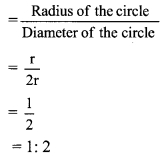∴ Ratio of radius to diameter of circle is 1 : 2.

ii. The ratio of diagonal to the length of a rectangle, having length 4 cm and breadth 3 cm.Let □ ABCD be a rectangle.
In ∆ABC, ∠B = 90°
AC2 = AB2 + BC2 … [Pythagoras theorem]
= 42 + 32 = 16 + 9
∴ AC2 = 25
AC = 5 … [Taking square root on both side]
The ratio of diagonal to the length of a rectangle = $$\frac { AC }{ AB }$$
= $$\frac { 5 }{ 4 }$$
= 5 : 4
∴ The ratio of diagonal to the length of a rectangle is 5 : 4

iii. The ratio of numbers denoting perimeter to area of a square, having side 4 cm. side of square = 4cm
Perimeter of square = 4 x side = 4 x 4 = 16 cm
Area of square = (side)2 = (4)2 = 16 cm2
Ratio of numbers denoting perimeter to area of square∴ The ratio of numbers denoting perimeter to area of a square is 1 : 1.

Question 4.
Check whether the following numbers are in continued proportion.
i. 2, 4, 8
ii. 1, 2, 3
iii. 9, 12, 16
iv. 3, 5, 8
Solution:
If a, b, c are in continued proportion then b2 = ac.
i. 2, 4, 8
Let, a = 2, b = 4 and c = 8
Here, b2 = 42 = 16
ac = 2 x 8 = 16
∴ b2 = ac
∴ 2, 4,8 are in continued proportion.

ii. 1, 2, 3
Let, a = 1, b = 2 and c = 3
Here, b2 = 22 = 4
ac = 1 x 3 = 3
∴ b2 ≠ ac
∴ 1, 2,3 are not in continued proportion.

iii. 9, 12, 16
Let, a = 9, b = 12 and c = 16
Here, b2 = 122 = 144
ac = 9 x 16 = 144
∴ b2 = ac
∴ 9, 12, 16 are in continued proportion.

iv. 3, 5, 8
Let, a = 3, b = 5 and c = 8
Here, b2 = 52 = 25
ac = 3 x 8 = 24
∴ b2 ≠ ac
∴ 3, 5, 8 are not in continued proportion.

Question 5.
a, b, c are in continued proportion. If a = 3 and c = 27, then find b.
Solution:
a, b, c are in continued proportion. …[Given]
∴ b2 = ac
∴ b2 = 3 x 27 …[∵ a = 3 and c = 27]
∴ b2 = 81
∴ b = 9 …[Taking square root of both sides]

Question 6.
Convert the following ratios into percentages.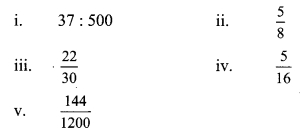Solution:
i. Let 37 : 500 = x%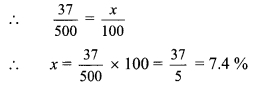∴ 37 : 500 = 7.4%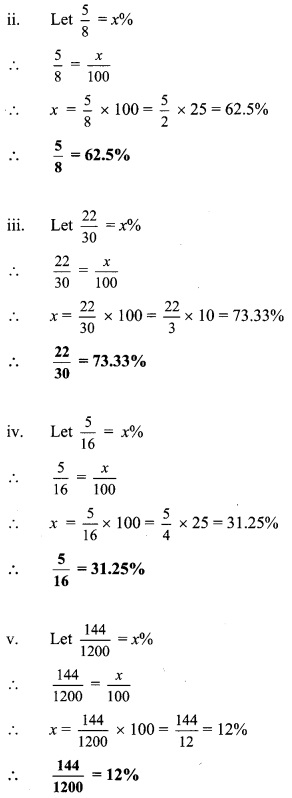Question 7.
Write the ratio of first quantity to second quantity in the reduced form.
i. 1024 MB, 1.2 GB [(1024 MB = 1GB)]
ii. ₹ 17, ₹ 25 and 60 paise
iii. 5 dozen, 120 units
iv. 4 sq.m, 800 sq.cm
v. 1.5 kg, 2500 gm
Solution:
i. 1024 MB, 1.2 GB
1024 MB = 1 GBii. ₹ 17, ₹ 25 and 60 paise
₹ 17 = 17 x 100 paise = 1700 paise
₹ 25 and 60 paise = (25x 100) paise + 60 paise
= (2500 + 60) paise
= 2560 paiseiii. 5 dozen, 120 units
5 dozen = 5 x 12 units = 60 unitsiv. 4 sq.m, 800 sq.cm
4 sq.m = 4 x 10000 sq.cm = 40000 sq.cmv. 1.5 kg, 2500 gm
1.5 kg = 1.5 x 1000 gm = 1500gm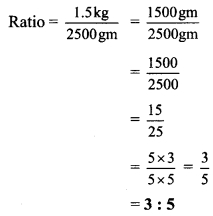Question 8.
If $$\frac { a }{ b }$$ = $$\frac { 2 }{ 3 }$$, then find the values of the following expressions.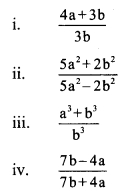Solution: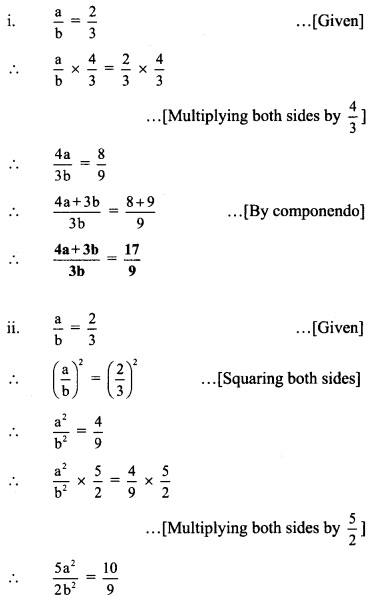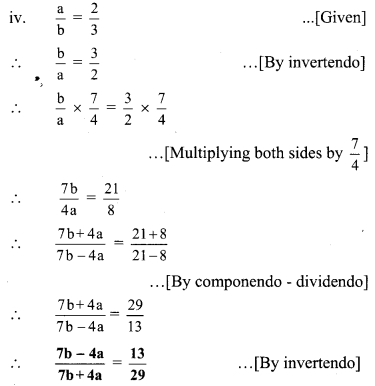Question 9.
If a, b, c, d are in proportion, then prove thatSolution:
a, b, c are in continued proportion. …[Given]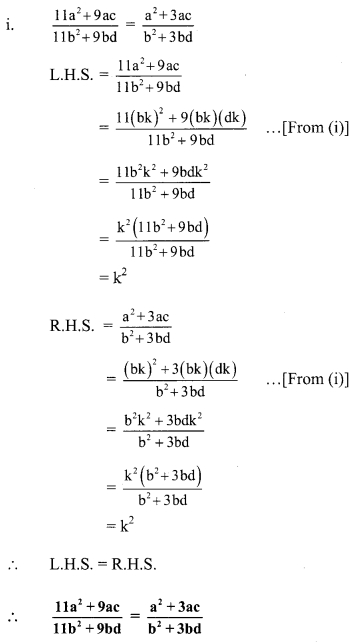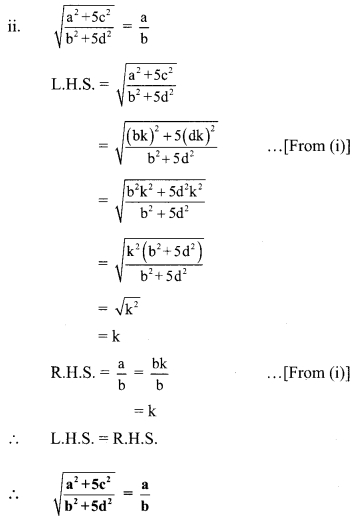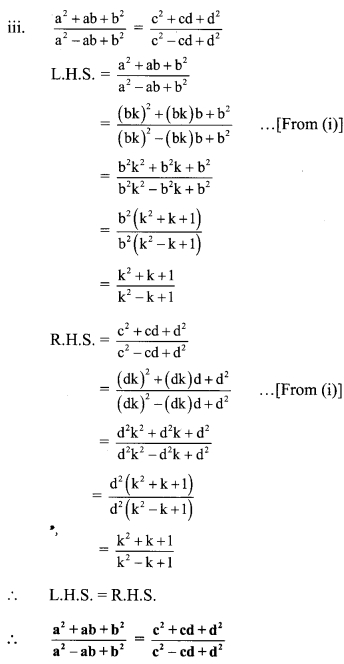Question 10.
If a, b, c are ¡n continued proportion, then prove that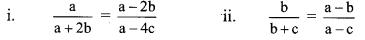Solution:
a, b, c are in continued proportion. … [Given]
∴ $$\frac { a }{ b }$$ = $$\frac { b }{ c }$$
Let $$\frac { a }{ b }$$ = $$\frac { b }{ c }$$ = k
∴ b = ck
∴ a = bk
= (ck)k . .. [From (j)]
a = ck2 …(ii)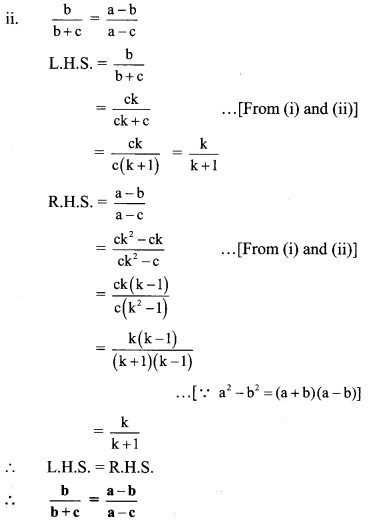Question 11.
Solve:
$$\frac{12 x^{2}+18 x+42}{18 x^{2}+12 x+58}=\frac{2 x+3}{3 x+2}$$
Solution: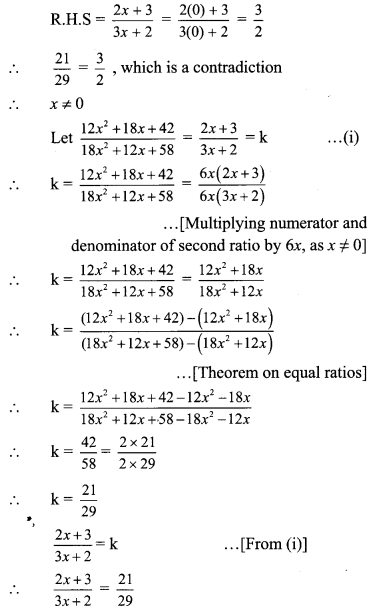∴ 29(2x + 3) = 21 (3x + 2)
∴ 5 + 87= 63x + 42
∴ 87 – 42 = 63x – 58x
∴ 45 = 5x
∴ x = 9
∴ x = 9 is the solution of the given equation.

Question 12.
If $$\frac{2 x-3 y}{3 z+y}=\frac{z-y}{z-x}=\frac{x+3 z}{2 y-3 x}$$ ,then prove that every ratio = $$\frac { x }{ y }$$.
Solution:Question 13.
If $$\frac{b y+c z}{b^{2}+c^{2}}=\frac{c z+a x}{c^{2}+a^{2}}=\frac{a x+b y}{a^{2}+b^{2}}$$, then prove $$\frac{x}{a}=\frac{y}{b}=\frac{z}{c}$$
Solution:Question 1.
Take 5 pieces of card paper. Write the following statements, one on each paper.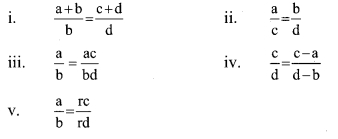a, b, c, d are positive numbers and $$\frac{a}{b}=\frac{c}{d}$$ is given. Which of the above statements are true or false, write at the back of each card, if false explain why. (Textbook pg. no. 70)
i. True
ii. True
iii. False
Here, numerator and denominator are multiplied by two different numbers a and b.
iv. False
Here, different numbers a and b are subtracted from numerator and denominator.
v. True

Question 2.
In the following activity, the values of a and b can be changed. That is by changing a : b we can create many examples. Teachers should give lot of practice to the students and encourage them to construct their own examples. (Textbook pg. no. 70)Question 3.
Observe the political map of India from a Geography text book. Study the scale of this map.
From the given scale find the straight line distances between various cities like
i. New Delhi to Bengaluru
ii. Mumbai to Kolkata
iii. Jaipur to Bhuvaneshvar. (Textbook pg. no. 77)
[Students should attempt the above activity on their own.]